## 线性支持向量机（Linear SVM）

### 最大间隔分类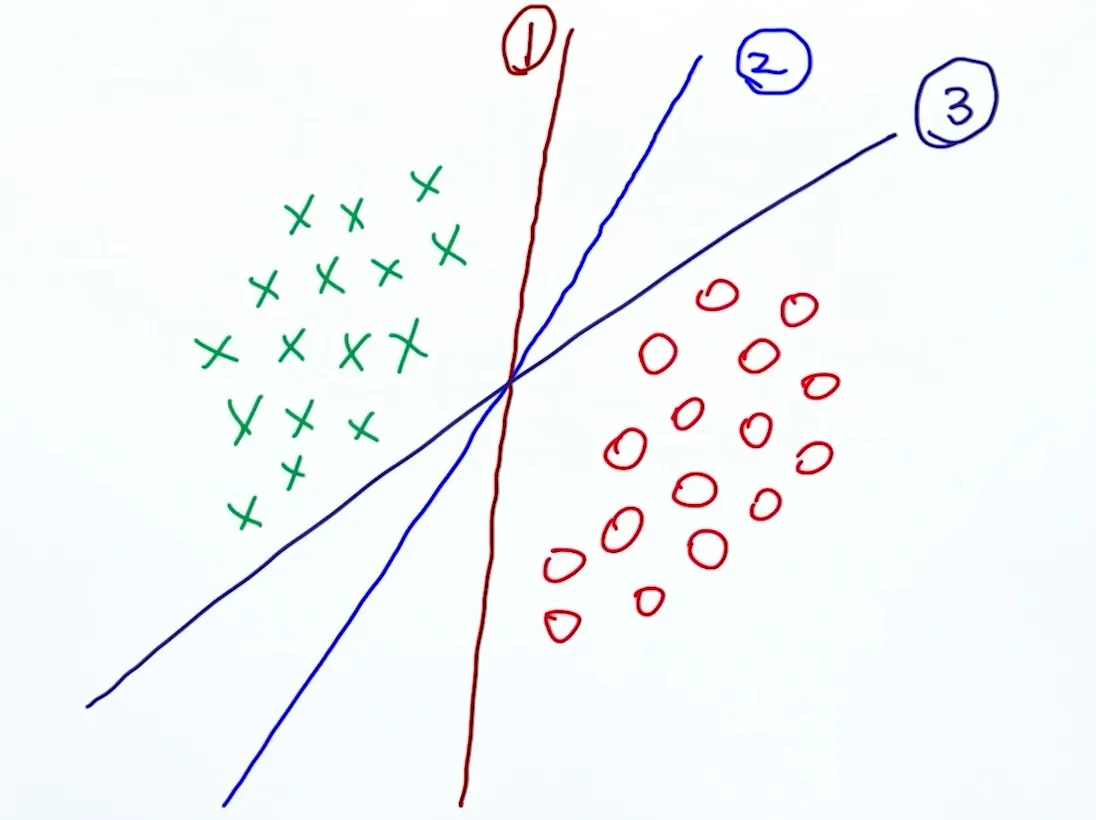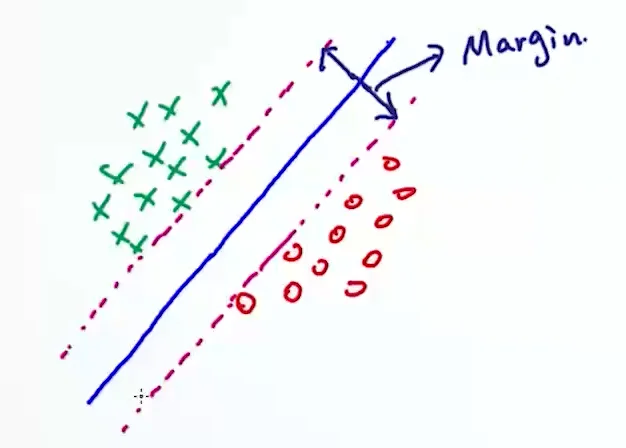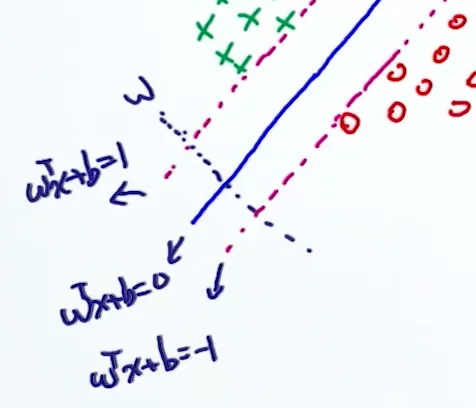$$x_+ = x_- + \lambda w$$ 代入 $$w^Tx_+ +b=1$$$w^T(x_- + \lambda w) +b=1 \\w^Tx_- + \lambda w^Tw +wb=1 \\\lambda w^Tw =2 \\\lambda = \frac{2}{w^Tw} \\$$$x_+ = x_- + \lambda w$$ 代入 margin 的表达式中： \begin{align*}\text{margin} &= |x_+ - x_-| \\&= |\lambda w| \\&= \lambda |w| \\&= \frac{2}{w^Tw} \cdot \| w \| \\&= \frac{2}{ \| w \|} \end{align*} 我们的目标就是让 margin 最大，也就是让 $$\frac {2} { \| w \| }$$ 最大。

### Hard Constraint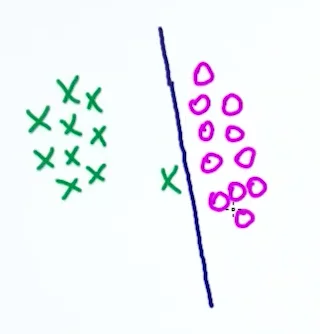### 目标求最优

• init $$w_0$$, $$b_0$$
• for $$i = 1...n$$
• if $$1-y_i (w^T x_i + b) \lt 0$$
• $$w^* = w - \eta_t \cdot 2w$$
• else
• $$w^* = w - \eta_t (2w+\lambda \frac{\part (1-y_i (w^T x_i + b))}{\part w})$$
• $$b^* = b - \eta_t(\lambda\frac{\part (1-y_i (w^T x_i + b))}{\part b})$$

### Linear SVM 的缺点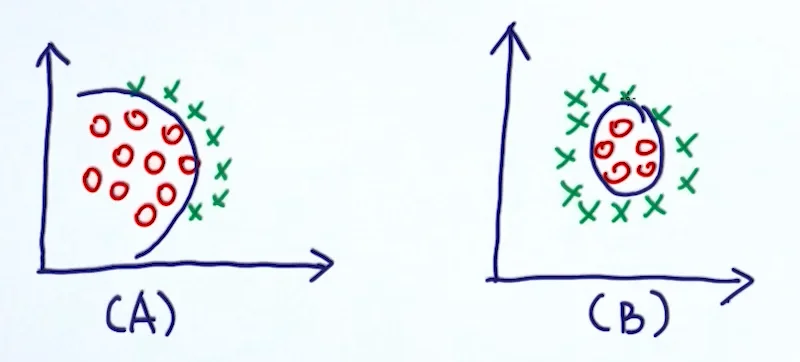• 利用非线性模型代替（比如神经网络）
• 把数据映射到高维空间，在高维空间学习线性模型

## Lagrangian: From Constrained To Unconstrained

### 单相等限制的情况处理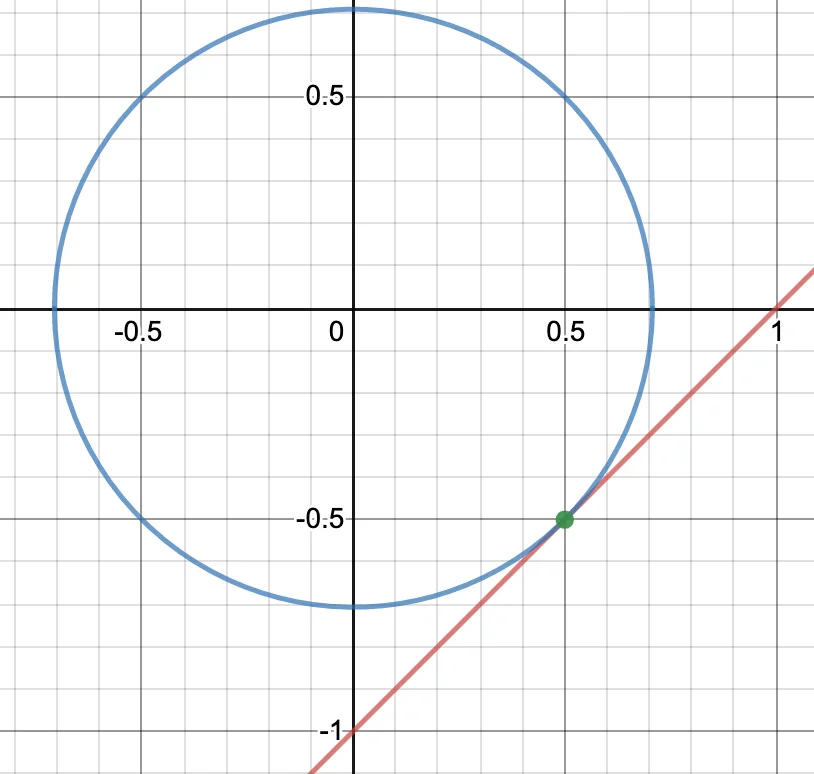$$x_1^2 + x_2^2$$ 表示的圆形与 $$x_2-x_1 = -1$$ 表示的直线相切，满足最小条件。此时，圆在点 $$(\frac{1}{2}, -\frac{1}{2})$$ 的切线与直线的斜率相等，即两条直线平行。

$$f(x)$$$$g(x)$$ 斜率相等，可以写成这种表达式： \begin{align*} \nabla_{x} f(x) = \pm \lambda \nabla_{x} g(x) \\ \nabla_{x} f(x) \mp \lambda \nabla_{x} g(x) = 0 \\ \nabla_{x}(f(x) \mp \lambda g(x)) = 0 \end{align*} 那也就是我们的变化式 $$\min f(x) + \lambda g(x)$$ 的导数等于 $$0$$。即： $\begin{array}{c}{\frac{\partial(f(x)+\lambda g(x))}{\partial x}=0} \\ {\frac{\partial(f(x)+\lambda g(x))}{\partial \lambda}=g(x)=0}\end{array}$

$$g(x)=0$$ 其实就是原始条件。

## Dual Derivation of SVM

### Primal Form vs Dual Form

1. Primal 问题有可能比较难解决
2. 在 Dual 问题上有可能会发现一些有趣的 insight

### Dual Form of SVM

$\frac{\partial L}{\partial b} = \sum_{i=1}^{n} \lambda_{i} y_{i}=0$

\begin{align*} \sum_{i=1}^n \lambda_i [1-y_i(w^Tx_i+b)] &= \sum_{i=1}^{n} \lambda_{i}-\sum_{i=1}^{n} \lambda_{i} \cdot y_{i}\left(w^Tx_i+b\right) \\ &=\sum_{i=1}^{n} \lambda_{i}-\sum_{i=1}^{n} \lambda_{i} y_{i} w^Tx_{i}-\sum_{i=1}^{n} \lambda_{i} y_{i}b \\ &=\sum_{i=1}^{n} \lambda_{i}-\sum_{i=1}^{n} \lambda_{i} y_{i} w^Tx_{i} \\ &=\sum_{i=1}^{n} \lambda_{i}-\sum_{i=1}^{n} \lambda_{i} y_{i} (\sum_{j=1}^{n} \lambda_{j} y_{j} x_{j})^Tx_{i} \\ &=\sum_{i=1}^{n} \lambda_{i}-\sum_{i=1}^{n} \sum_{j=1}^{n} \lambda_{i} \lambda_{j} y_{i} y_{j} x_i^T x_j \end{align*}

## Kernel Trick

### Kernel Trick

Kernel Trick 其实不仅仅针对 SVM。只要目标函数里有这么一个内积的项，就可以使用。它有很多种形式——

• Linear Kernel: $$K(x,y) = x^T y$$
• 数据样本非常多时，用 Linear Kernel 效果会很好。
• Polynomial Kernel: $$K(x,y) = (1+x^Ty)^d$$
• Gaussian Kernel: $$K(x,y)=\exp(\frac{-\|x-y\|^2}{2\sigma^2})$$